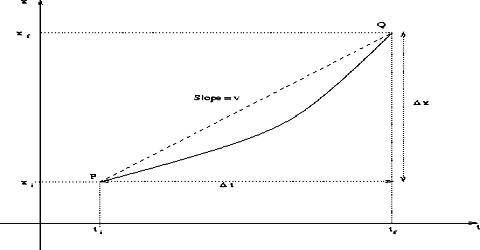Physics

# Average Velocity Related to MotionAverage velocity: The quantity which is obtained by dividing the total displacement by time taken for that displacement is called average velocity. It can be defined as the displacement divided by the time.

Explanation: Let total displacement of a body be ∆r in time interval of ∆t.

So, Average velocity, v = ∆r/∆t

If the motion is one dimensional and if the body is in motion along X-axis, then there exists only one component of velocity. The component will be-

vx = ∆x/∆t î   [in one-dimensional reference, r = xî)

and magnitude of average velocity, vx = ∆x/∆t

Example:

First we require estimating the net displacement of an object for the entire motion to find the average velocity. The initial direction of movement of the object is normally taken as reference direction.Suppose a particle travels a distance d1 in a time t1, d2 in a time t2, and d3 in a time t3, as shown above. It may be noted that the distances traveled are not in the same directions. The total distance is d1 + d2 + d3, but it is not the net displacement. The net displacement is the projections of d2 and d3, in the direction of d1, are d2 + d3 and hence the net displacement is d1 + d2 + d3. Therefore, in the above case the average velocity is found as,

vavg = [d1 + d2 + …. dn/ t1 + t2 + …..tn]

It may be noted that at any time, if the velocity is in the direction of an obtuse angle to the reference direction then the projection of that particular velocity will be negative.